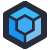These days, every language in wide use has support for unicode characters, at least in strings. This is excellent, because it means we can program like the kids these days, with loads of emojis. I'm cool. This is what cool looks like: 😎.

You know what else is cool? National ID systems. One particular European country has rolled out a new software backend for their national ID system. Dimitry has the privilege to see the code. This is how they show the user how complete something is, as a percentage:

``````private static string GetPercentageRounds(double percentage)
{
if (percentage == 0)
return "⚪⚪⚪⚪⚪⚪⚪⚪⚪⚪";
if (percentage > 0.0 && percentage <= 0.1)
return "🔵⚪⚪⚪⚪⚪⚪⚪⚪⚪";
if (percentage > 0.1 && percentage <= 0.2)
return "🔵🔵⚪⚪⚪⚪⚪⚪⚪⚪";
if (percentage > 0.2 && percentage <= 0.3)
return "🔵🔵🔵⚪⚪⚪⚪⚪⚪⚪";
if (percentage > 0.3 && percentage <= 0.4)
return "🔵🔵🔵🔵⚪⚪⚪⚪⚪⚪";
if (percentage > 0.4 && percentage <= 0.5)
return "🔵🔵🔵🔵🔵⚪⚪⚪⚪⚪";
if (percentage > 0.5 && percentage <= 0.6)
return "🔵🔵🔵🔵🔵🔵⚪⚪⚪⚪";
if (percentage > 0.6 && percentage <= 0.7)
return "🔵🔵🔵🔵🔵🔵🔵⚪⚪⚪";
if (percentage > 0.7 && percentage <= 0.8)
return "🔵🔵🔵🔵🔵🔵🔵🔵⚪⚪";
if (percentage > 0.8 && percentage <= 0.9)
return "🔵🔵🔵🔵🔵🔵🔵🔵🔵⚪";

return "🔵🔵🔵🔵🔵🔵🔵🔵🔵🔵";
}
``````

Sure, this is an ugly way to generate strings, but the real secret sauce is the first comparison: `percentage == 0`. That's fine in some places, like when you know `percentage` was initialized to `0`, but when it's being calculated, it could easily be very close to zero without actually being zero, thanks to a loss of precision. That's why the `Equals` and `CompareTo` functions should be used, otherwise some user is going to be at 0% and see a completed progress bar.[Advertisement] ProGet’s got you covered with security and access controls on your NuGet feeds. Learn more.